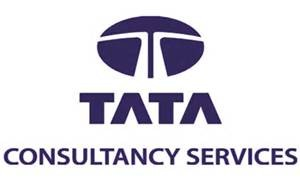21) A red house is built from red bricks. A blue house is built from blue bricks. A white house is built from white bricks. Then what is a greenhouse made from?
Explanation:
Glass i.e. Buildings are made of bricks. But a greenhouse is made of glass.
22) If Rs. 782 be divided into three parts, proportional to 1/2: 2/3: 3/4, then the first part is:
Explanation:
Given ratio = 1/2: 2/3:3/4 = 6: 8: 9 1st part = 782*(6/22) = 213.2727.. Approximately equals to 213 www.allindiaobs.in

23) A man decides to buy a nice horse. He pays \$60 for it, and he is very content with the strong animal. After a year, the value of the horse has increased to \$70 and he decides to sell the horse . But after a few days later he regrets his decision to sell the beautiful horse, and he buys it again. Unfortunately he has to pay \$80 to get it back, so he loses \$10. After another year of owing the horse, he finally decides to sell the horse for \$90. What is the overall profit the man makes?
Explanation:
The total expenses are \$60 + \$80 = \$140 and the total earnings are \$70 +\$90 = \$160. The overall profit = \$160 – \$140 = \$ 20

24) When I become as old as my father is now, I will be 5 times the age of my son. In addition, my son will be 8 yrs older than What I am now. If the sum of my father’s age and my age is equal to100. How old is my son now?
Explanation:
Let us consider the ages of the man, his father & his son is m, f & s respectively. Then according to the problem F=5s………… (i) S + f-m = m+8…….. (ii) F + m = 100……….. (iii) Putting (i) in (iii) we get 5s+m=100…….. (iv) Putting (i) in (ii) we get 6s-2m=8………… (v) Equating (iv) & (v) we get s = 13, f = 65 & m = 36

25) Nine persons went to the hostel for taking their meals, 8 of them spent Rs.12 each on their meals and ninth spend Rs. 8 more then the average expenditure of all nine. What is the total money spent by them?
Explanation:
Let average expenditure be x Then 8*12 +(x+8) = 9x i.e. 104 + x = 9x; 8x = 104 x = 13 Therefore total money spent is 9x = 9*13 =117

26) The average of a list of 6 numbers is 20. If we remove one of the numbers, the average of the remaining numbers is 15. What is the number that was removed?
Explanation:
The removed number could be obtained by difference between the sum of original 6 numbers and the sum of remaining 5 numbers i.e. sum of original 6 numbers – sum of remaining 5 numbers sum of original 6 numbers = 20 × 6 = 120 sum of remaining 5 numbers = 15 × 5 = 75 Number removed = sum of original 6 numbers – sum of remaining 5 numbers 120 – 75 = 45

27) In the word ORGANISATIONAL, if the first and second, third and fourth, fifth and sixth letters are interchanged, what would be the 12th letter from right?
Explanation:
After interchanging R O A G I N S A T I O N A L 14 13 12 11 10 9 8 7 6 5 4 3 2 1

28) What are the number of edges, number of vertices and number of faces of a planar cube among the following options?
We know cube has 6 faces, 12 edges and 8 vertices

29 How many alphabets need to be there in a language if one were to make 1 million distinct 3 digit initials using the alphabets of the language?
Explanation:
Let the number of required alphabets in the language be ‘n’. Therefore, using ‘n’ alphabets we can form n * n * n = n3 distinct 3 digit initials. These n3 different initials = 1 million i.e. n3 = 106 (1 million = 106) => n3 = (102)3 => n = 102 = 100

30) Today’s high school students spend too much time thinking about trivial and distracting matters such as fashion. Additionally, they often dress inappropriately on school grounds. Rather than spending time writing another detailed dress policy, we should make school uniforms mandatory. If students were required to wear uniforms, it would increase a sense of community and harmony in our schools and it would instill a sense of discipline in our students. Another positive effect would be that teachers and administrators would no longer have to act as clothing police, freeing them up to focus on more important issues.
This paragraph best supports the statement that
Options: